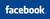AllBankingSolutions.com ......our answer to all your banking needs

 Follow AllBankingSolutions  @EMI CALCULATOR IN EXCEL

Ads by Google

Now a days Excel is very popular and frequently people use it for calculations.  People who are familiar with EXCEL can use.  PMT formula is used to know the EMI.  IPMT is used to know the interest portion in the EMI of a particular instalment.  Similarly, PPMT is used to know the principal component in the EMI of a particular installment.  We give below the details as to how to use PMT formula in excel.

PMT                                                                                                                         (Source : EXCEL Help File)

Calculates the payment for a loan based on constant payments and a constant interest rate.

Syntax

PMT(rate,nper,pv,fv,type)

For a more complete description of the arguments in PMT, see the PV function.

Rate   is the interest rate for the loan.

Nper   is the total number of payments for the loan.

Pv   is the present value, or the total amount that a series of future payments is worth now; also known as the principal.

Fv   is the future value, or a cash balance you want to attain after the last payment is made. If fv is omitted, it is assumed to be 0 (zero), that is, the future value of a loan is 0.

Type   is the number 0 (zero) or 1 and indicates when payments are due.

 Set type equal to If payments are due 0 or omitted At the end of the period 1 At the beginning of the period

Remarks

• The payment returned by PMT includes principal and interest but no taxes, reserve payments, or fees sometimes associated with loans.
• Make sure that you are consistent about the units you use for specifying rate and nper. If you make monthly payments on a four-year loan at an annual interest rate of 12 percent, use 12%/12 for rate and 4*12 for nper. If you make annual payments on the same loan, use 12 percent for rate and 4 for nper.

Tip  To find the total amount paid over the duration of the loan, multiply the returned PMT value by nper.

.

Ads by Google

Example 1

The example may be easier to understand if you copy it to a blank worksheet.

1. Create a blank workbook or worksheet.
2. Select the example in the Help topic.

Note  Do not select the row or column headers.

Selecting an example from Help

1. Press CTRL+C.
2. In the worksheet, select cell A1, and press CTRL+V.
3. To switch between viewing the results and viewing the formulas that return the results, press CTRL+` (grave accent), or on the Tools menu, point to Formula Auditing, and then click Formula Auditing Mode.
 1 2 3 4

 A B Data Description 8% Annual interest rate 10 Number of months of payments 10000 Amount of loan Formula Description (Result) =PMT(A2/12, A3, A4) Monthly payment for a loan with the above terms (-1,037.03) =PMT(A2/12, A3, A4, 0, 1) Monthly payment for a loan with the above terms, except payments are due at the beginning of the period (-1,030.16)

Example 2

You can use PMT to determine payments to annuities other than loans.

The example may be easier to understand if you copy it to a blank worksheet.

1. Create a blank workbook or worksheet.
2. Select the example in the Help topic.

Note  Do not select the row or column headers.

Selecting an example from Help

1. Press CTRL+C.
2. In the worksheet, select cell A1, and press CTRL+V.
3. To switch between viewing the results and viewing the formulas that return the results, press CTRL+` (grave accent), or on the Tools menu, point to Formula Auditing, and then click Formula Auditing Mode.
 1 2 3 4

 A B Data Description 6% Annual interest rate 18 Years you plan on saving 50,000 Amount you want to have save in 18 years Formula Description (Result) =PMT(A2/12, A3*12, 0, A4) Amount to save each month to have 50,000 at the end of 18 years (-129.08)

Note  The interest rate is divided by 12 to get a monthly rate. The number of years the money is paid out is multiplied by 12 to get the number of payments.

 You can give your feedback / comments about this Article.   Please give only relevant comments as irrelevant comments are waste of time for yourself and our other readers.    Please enable JavaScript to view the comments powered by Disqus. blog comments powered by Disqus